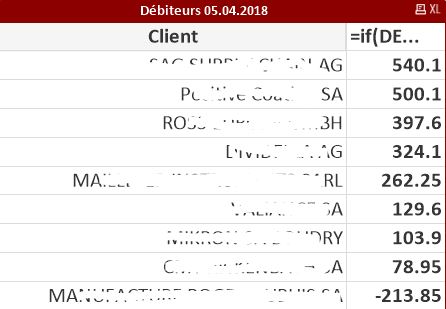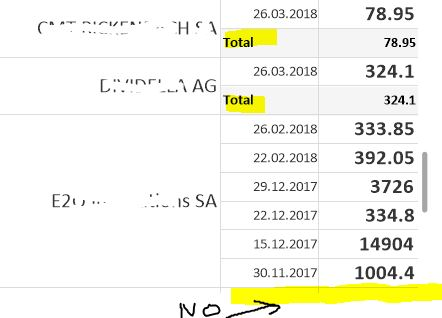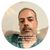# QlikView App Dev

Discussion Board for collaboration related to QlikView App Development.

Announcements
Action-Packed Learning Awaits! QlikWorld 2023. April 17 - 20 in Las Vegas: REGISTER NOW
cancel
Showing results for
Did you mean:Creator

## Sum sales between specific dates

Hello !

I'm stuck trying to displaying the sum of money that are due from our clients and not yet paid, but for specific dates

Every Week, 1 person has to export a list from our ERP to Excel.

This file is then used in QlikView (charts, ...)

The tables are

CLIENT        DOCUMENT NBR          DUE DATE          AMOUNT

A                    12345-1                        03.04.2018              50.-

A                    14578-2                        07.05.2018            100.-

B                    25478-7                        04.04.2018            100.-

C                    58485-1                       09.05.2018               50.-

TOTAL                                                               300.-

I'd like to show the total amount due for each client, only if the DUE DATE is <=Date(Today())

If i use today's date as example 05.04.2018 (DD.MM.YYYY), my table should look like this:

CLIENT        DOCUMENT NBR          DUE DATE          AMOUNT

A                    12345-1                        03.04.2018              50.-

TOTAL A                                                                            50.-

B                    25478-7                        04.04.2018            100.-

TOTAL  B                                                                          100.-

TOTAL:                                                              150.-

Actually, I'd like to make the simplest table >

CLIENT                  AMOUNT

A                                  50.-

TOTAL A                      50.-

B                                100.-

TOTAL  B                  100.-

TOTAL:                150.-

I tried the following expression

=if(DUE_DATE <= Date(Today()), sum(AMOUNT))

Here's what my table should look like (using manual date selection)and this is what it looks like if I use my expressionAs you can see, it seems to be working as some values are correct (103.90, 129.60, 540.10, ...)

but if I have more than 1 DUE_DATE for a CLIENT, it does not show me the total amount

Every Client that don't show have multiple dates and amount

As you can see here, the total is not displayed if I use another tableThank you for your help !

FG

1 Solution

Accepted SolutionsMVP

You may try this -

=Sum(if(DUE_DATE <= Date(Today()), AMOUNT))

6 RepliesContributor II

I suggest making a custom dimension that is every date that is past due.
Then check show subtotals in presentation tab.
edit----
it seems that your sales expression is a dimension.
Try sum(AMOUNT) even though it's 1-1 granular in expression instead of just the dimension nameMVP

You may try this -

=Sum(if(DUE_DATE <= Date(Today()), AMOUNT))MVP

Or may be using set expression -

Sum({<DUE_DATE={"<=\$(=Date(Today()))"}>}AMOUNT)Master III

May be try

Sum({<DUE_DATE={"<=\$(=Date(toDay(),'DD.MM.YYYY'))"}>} AMOUNT)Master III

If the expression does not work, pl provide a sampleCreator
Author

Sorry for my late reply !

You solution works, Thank you a lot Digvijay !!Best wishesCommunity Browser